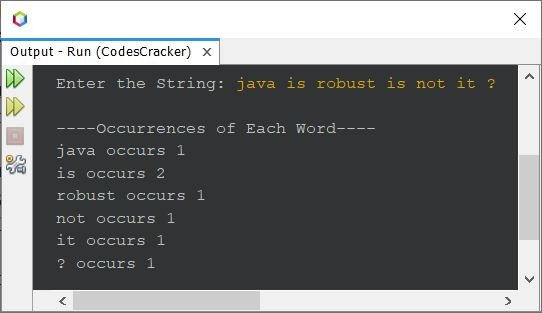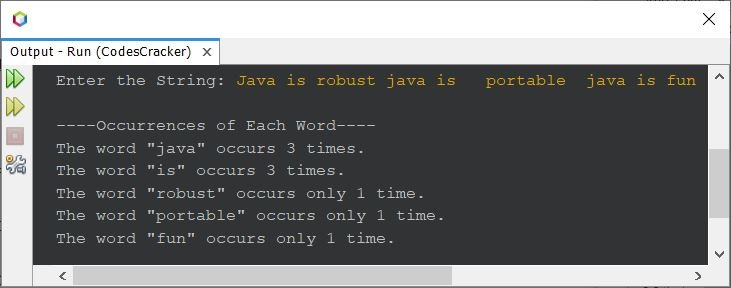# Java Program to Count the Occurrence of Each Word in a Given String

## Count Each Word in Given String - Basic Version

The question is, write a Java program to find and print the frequency of each word in a string. The string must be received by user at run-time of the program. The answer to this question, is the program given below:

```import java.util.Scanner;

public class CodesCracker
{
public static void main(String[] args)
{
String str, word;
int wordsLen, i, count, j, k;
Scanner s = new Scanner(System.in);

System.out.print("Enter the String: ");
str = s.nextLine();

String words[] = str.split(" ");
wordsLen = words.length;

System.out.println("\n----Occurrences of Each Word----");
for(i=0; i<wordsLen; i++)
{
word = words[i];
count = 1;
for(j=(i+1); j<(wordsLen-1); j++)
{
if(word.equals(words[j]))
{
count++;
for(k=j; k<(wordsLen-1); k++)
{
words[k] = words[k+1];
}
wordsLen--;
j--;
}
}
System.out.println(word+ " occurs " +count);
count = 0;
}
}
}```

The snapshot given below shows the sample run of above Java program on finding and printing the frequency of each word in a given string, with user input java is robust is not it ? as string:## Count Each Word in Given String - Complete Version

Since there are some limitations with above program, like the same word with character(s) of lowercase and uppercase will get treated as different word, multiple white spaces are not allowed. Also the output looks basic. Therefore I've modified that program and created a new one. Here is the program after modifying above one.

```import java.util.Scanner;

public class CodesCracker
{
public static void main(String[] args)
{
Scanner s = new Scanner(System.in);

System.out.print("Enter the String: ");
String str = s.nextLine();

str = str.toLowerCase();
String words[] = str.split("\\s+");
int wordsLen = words.length;

System.out.println("\n----Occurrences of Each Word----");
for(int i=0; i<wordsLen; i++)
{
int count = 1;
for(int j=(i+1); j<(wordsLen-1); j++)
{
if(words[i].equals(words[j]))
{
count++;
for(int k=j; k<(wordsLen-1); k++)
words[k] = words[k+1];
wordsLen--;
j--;
}
}
if(count==1)
System.out.println("The word \"" +words[i]+ "\" occurs only 1 time.");
else
System.out.println("The word \"" +words[i]+ "\" occurs " +count+" times.");
count = 0;
}
}
}```

Here is its sample run with user input Java is robust java is portable java is fun as string to find and print the occurrences of each word available in it. In between the word is and portable, there are 3 white spaces. And in between the word portable and java, there are two white spaces:Java Online Test

« Previous Program Next Program »